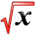# AntiderivativeThis article is part of the MathHelp Tutoring Wiki

## Definition

Given a function $f$, we say that a function $F$is an antiderivative of $f$if $F'(x)=f(x)$for all values of $x$.

#### Example 1

If $f(x)=x^{2}$, the antiderivative of $f$is $F(x)=x^{3}/3+c$where $c$can be any real number.

#### Example 2

If $f(x)=1/x$, the antiderivative of $f$is $F(x)=\ln(x)+c$where $c$can be any real number.

## Physical interpretation

The derivative of distance is velocity. The derivative of velocity is acceleration

#### Example

A boy throws a baseball up in the air from the edge of a cliff 400 ft above the ground. The initial speed of the ball as it leaves his hand is 40 ft/sec. When does the ball reach its maximum height? Note: g (acceleration due to gravity) is 32 ft/s2

#### Solution

We know that the acceleration is -32ft/s2 a(t)=dv/dt=-32

So by taking the antiderivative: v(t)= -32(t)+C

Now we need to determine what the constant C is. To do this, we set t=0.

v(0)=40 so 40=0+C, so v(t)= -32t+40

To find the maximum height reached by the ball, set v(t)=0 to find the time at which it reaches this point

0= -32(t)+40

32t=40

t= 4/5 seconds

since s'(t)=v(t), we must antidifferentiate again

s(t)= -16t2 +40t+D

Now we must determine what the constant D is. To do this, we set t=0

s(0)= 400

So, s(t)= -16t2 +40t +400

When the ball hits the ground, s(t)=0

-16t2 +40t+400=0

-2t2+5t+50=0

2t2-5t-50=0

Solve for t.